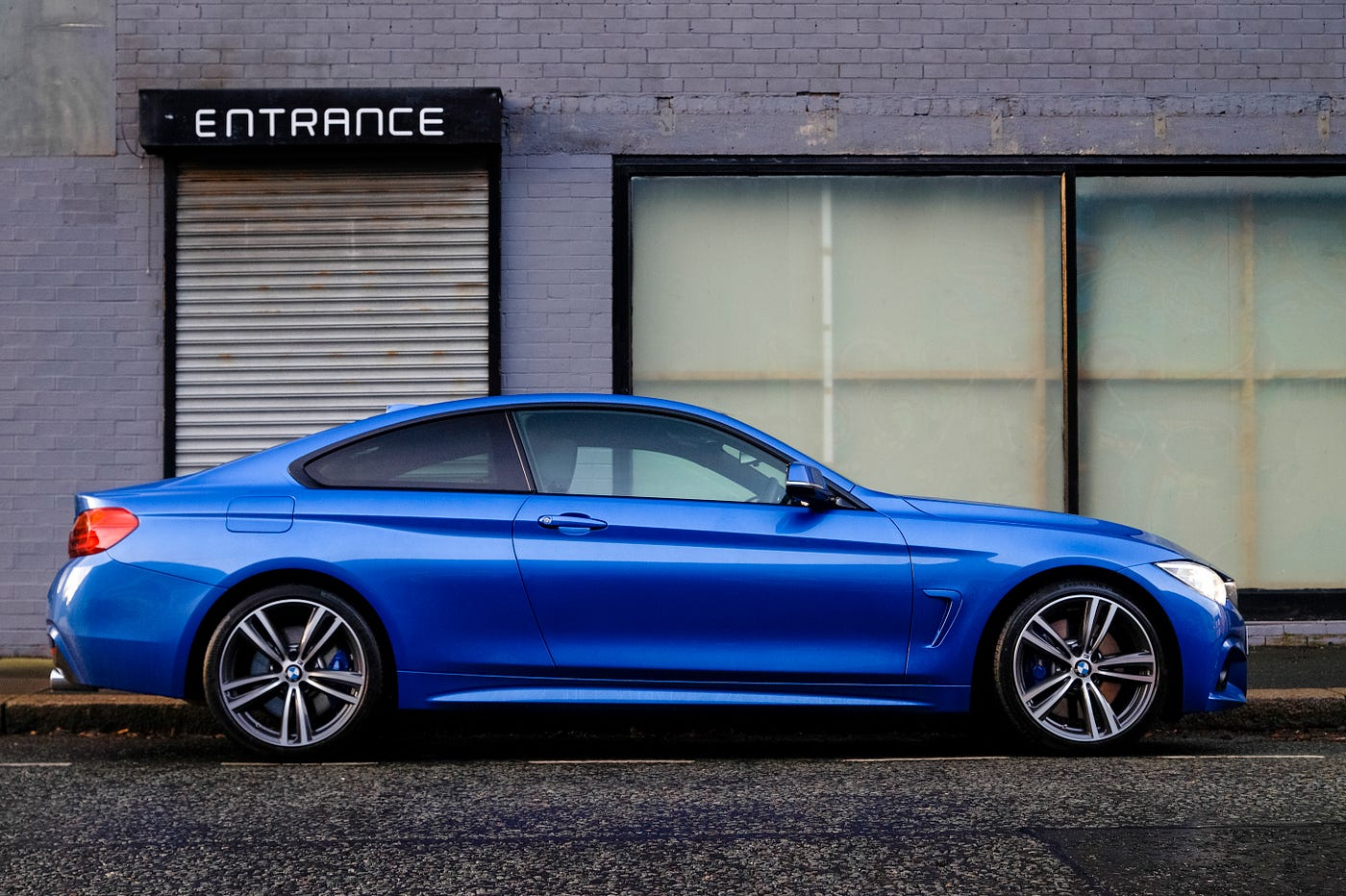# A primer into Autonomous Vehicle- From Controls Perspective — Part 1

I will keep this story short and crisp so that even a novice reader gets an idea of what goes behind a self-driving car. So let’s dive straight into it.

What’s a PID and why we need it?

It’s a control algorithm that lets your system follow the desired command— Found in almost all industrial applications. The basic idea behind a PID controller is to set/tune the Proportional, Derivative, and Integral gains(AKA constants). It provides the ability to automatically maintain the set value and compensate for changes in process and environment.

Here’s what a typical structure of PID looks like.

A block diagram of PID control system having feedback closed loop. Here τ(t) is the setpoint or desired value while y(t) is the value of the variable known as a process variable (PV). The value of PV is calculated as PV = y(t) : e(t) = τ(t) -y(t), and the corrective action is applied based on the proportional, integration and deviation rules. This controller takes corrective actions by adjusting the control variable (CV) u(t).

To understand more about the effect of each of these gains and how they reduce the error in the system, I recommend you read this link.

Problem Statement

Our autonomous vehicle must follow a certain trajectory/path. We consider a case of a car and divide the problem into two parts:

Longitudinal Control & Lateral Control

# Longitudinal Control:

We make sure that we can control the velocity of the car by letting it follow a predefined velocity profile. We assume that there is no offset/cross-track error, meaning that our vehicle is exactly following the path intended. We are interested only in the velocity with which the car must accelerate in order to reach the desired position. A very basic idea is to make the speed of the car slower where the curvature of the path is larger and vice-versa.

# Lateral Control:

In lateral control, we wish to remove the cross-track error that is with respect to the desired trajectory. In the case of lateral control, the reference signal is always zero, because we want our car to exactly match the path.

We can divide the lateral control into three more control techniques that take into account the kinematics of the system, which we previously skipped.

The names of these control algorithms are Pure Pursuit, Stanley, and MPC controller.

# Pure Pursuit Controller

Pure pursuit is the geometric path tracking controller. A geometric path tracking controller is any controller that tracks a reference path using only the geometry of the vehicle kinematics and the reference path. Pure Pursuit controller uses a look-ahead point which is a fixed distance on the reference path ahead of the vehicle as follows. The vehicle needs to proceed to that point using a steering angle which we need to compute.

The target point is chosen as the red point in the above figure. And the distance between the rear axle and the target point is denoted as ld ( Look-ahead Distance). Our target is to make the vehicle steer at a correct angle and then proceed to that point. So the geometric relationship figure is as follows, the angle between the vehicle’s body heading and the look-ahead line is referred to as α. Because the vehicle is a rigid body and proceeds around the circle. The instantaneous center of rotation(ICR) of this circle is shown as follows and the radius is denoted as R.

# Pure Pursuit Formulation

From the law of sines:

k is the curvature. Using the bicycle model (If you have no idea about the kinematic bicycle model, you can refer to another article named “Simple Understanding of Kinematic Bicycle Model”),

So the steering angle δ can be calculated as:

The pure pursuit controller is a simple control. It ignores dynamic forces on the vehicles and assumes the no-slip condition holds at the wheels. Moreover, if it is tuned for low speed, the controller would be dangerously aggressive at high speeds. One improvement is to vary the look-ahead distance ld based on the speed of the vehicle.

So the steering angle changed as:

In my next article, I will explain Stanley and MPC controller. More discussion on MPC controllers and fundamentals for designing a MPC controller will be explained.Photo by Grahame Jenkins on Unsplash

--

--